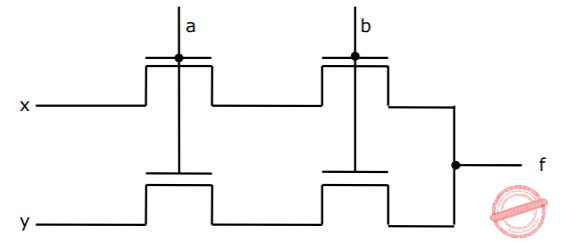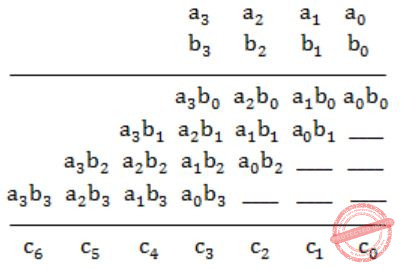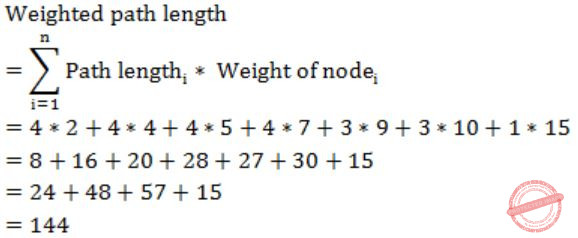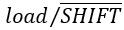## GATE 1991

 Question 1

For the digital in figure, the expression for the output f is ________A Out of syllabus.
Digital-Logic-Design       Circuits
 Question 2

In interleaved memory organization, consecutive words are stored in consecutive memory modules in ________ interleaving, whereas consecutive words are stored within the module in ________ interleaving.

 A low order, high order
Computer-Organization       Memory-Organization
Question 2 Explanation:
→ Consecutive words in consecutive memory modules in low order interleaving as the lower order bits determine the module.
→ Consecutive words in same memory module in high order interleaving as the higher order bits determine the module.
 Question 3

Consider the number given by the decimal expression:

`  163 * 9 + 162 * 7 + 16 * 5 + 3`

The number of 1’s in the unsigned binary representation of the number is ________.

 A 9
Digital-Logic-Design       Number-Systems
Question 3 Explanation:
Hexadecimal representation of a given no. is,
(9753)16
It's binary representation is,
1001011101010011
∴ The no. of 1's is 9.
 Question 4

Using the 8087 arithmetic coprocessor with the 8087 CPU requires that the 8087 CPU is operated ________.

 A Out of syllabus.
Computer-Organization       Microprocessor
 Question 5

When two 4-bit binary number A = a3a2a1a0 and B = b3b2b1b0 are multiplied, the digit c1 of the product C is given by _________

 A c1 = b1a0 ⊕ a1b0
Digital-Logic-Design       Number-Systems
Question 5 Explanation:⇒ c1 = b1a0 ⊕ a1b0
 Question 6

Consider the following PASCAL program segment:

``` if i mode 2 = 0 then
while i > = 0 do
begin
i:=i div 2;
if i mod 2 < > 0 do then i:=i – 1
else i:=i – 2
end```

An appropriate loop-invariant for the while-loop is ______

 A PASCAL is out of syllabus.
Programming       PASCAL-Programming
 Question 7

The minimum number of comparisons required to sort 5 elements is _____

 A 7
Algorithms       Sorting
Question 7 Explanation:
Minimum no. of comparisons
= ⌈log(n!)⌉
= ⌈log(5!)⌉
= ⌈log(120)⌉
= 7
 Question 8

The weighted external path length of the binary tree in figure is _____A 144
Algorithms       Binary-Trees
Question 8 Explanation:Question 9

If the binary tree in figure is traversed in inorder, then the order in which the nodes will be visited is ______A 4, 1, 6, 7, 3, 2, 5, 8
Algorithms       Tree Traversals
Question 9 Explanation:
Inorder traversal is
(Left, Root, Right)
So, the order will be
4, 1, 6, 7, 3, 2, 5, 8
 Question 10

Consider the following recursive definition of fib:

``` fib (n) : = if n = 0 then 1
else if n = 1 than 1
else fib (n – 1) + fib (n – 2)```

The number of times fib is called (including the first call) for an evaluation of fib (7) is ___________

 A 41
Programming       Recursion
Question 10 Explanation:
The recurrence relation for the no. of calls is
T(n) = T(n-1) + T(n-2) + 2
T(0) = T(1) = 0 (for fib(0) and fib(1), there are no extra recursive calls)
T(2) = 2
T(3) = 4
T(4) = 8
T(5) = 14
T(6) = 24
T(7) = 40
Counting the initial call, we get
40+1 = 41
 Question 11

The arithmetic expression : (a + b) * c - d / e * * f is to be evaluated on a two-address machine, where each operands, the number of registers required to evaluate this expression is ______. The number of memory access of operand is __________.

 A 3, 4
Compiler-Design       Operands
Question 11 Explanation:
** is used for exponentiation.
So, in total 3 registers are required and 6 memory operations in total to fetch all operands.
 Question 12

A given set of processes can be implemented by using only parbegin/parend statement, if the precedence graph of these processes is ________

 A properly nested.
Compiler-Design       Precedence-Graph
Question 12 Explanation:
A given set of processes can be implemented by using only parbegin/parend statement, if the precedence graph of these processes is properly nested.
 Question 13

The number of integer-triples (i.j.k) with 1 ≤ i.j.k ≤ 300 such that i + j + k is divisible by 3 is ________

 A Fill in the blanks.
Engineering-Mathematics       Linear-Algebra
 Question 14

If the longest chain in a partial order is of length n then the partial order can be written as a ______ of n antichains.

 A disjoint
Engineering-Mathematics       Set-Theory
Question 14 Explanation:
Suppose the length of the longest chain in a partial order is n. Then the elements in the poset can be partitioned into a disjoint antichains.
 Question 15

The maximum number of possible edges in an undirected graph with a vertices and k components is ________.

 A (n-k)(n-k+1)/2
Engineering-Mathematics       Graph-Theory
Question 15 Explanation:
N vertices and K components.
To get maximum, take one vertex each for each component, except last component.
Now, k-1 components have 1 vertex each and so on edges.
The last component has (n-(k-1)) vertices. So make the last component complete, i.e., it has n-(n-1)C2
= (n-k)(n-k+1)/2
= maximum no. of edges
 Question 16

Match the pairs in the following questions by writing the corresponding letters only.

```(A) IEEE 488    (P) specifies the interface for connecting a single device
(B) IEEE 796    (Q) specifies the bus standard for connecting a computer to
other devices including CPU’s
(C) IEEE 696    (R) specifies the standard for an instrumentation bus
(D) RS232-C     (S) specifies the bus standard for the “backplane” bus called
multibus.
```
 A Out of syllabus.
Computer-Networks       General
 Question 17

Match the pairs in the following questions by writing the corresponding letters only. For the 8086 microprocessor:

```(A) RQ/GT   (P) Used by processor for holding the bus for consecutive instruction
cycles.
(B) LOCK    (Q) Used for extending the memory or I/O cycle times
(C) HOLD    (R) Used for getting hold of processor bus in minimum bus mode
(D) READY   (S) Used for requesting processor bus in minimum bus mode.```
 A Out of syllabus.
Computer-Organization       Microprocessor
 Question 18

Match the pairs in the following questions by writing the corresponding letters only.

```(A) Buddy system     (P) Run-time type specification
(B) Interpretation   (Q) Segmentation
(C) Pointer type     (R) Memory allocation
(D) Virtual memory   (S) Garbage collection```
 A A-R, B-P, C-S, D-Q
Operating-Systems       General
Question 18 Explanation:
A-R
B-P
C-S
D-Q
Buddy system: The buddy system is a technique to allocate the memory by dividing the memory into partitions to try to satisfy a memory request as suitably as possible.
Interpretation: Runtime.
Pointer type: Garbage collector dereference the dangling pointer.
Virtual memory: Segmentation.
 Question 19

Match the pairs in the following questions by writing the corresponding letters only.

```(A) The number distinct binary trees with n nodes       (P) n!/2
(B) The number of binary strings of length of 2n        (Q) (3n/n)
with an equal number of 0’s and 1’s
(C) The number of even permutations of n objects        (R) (2n/n)
(D) The number of binary strings of length 6m which     (S) 1/n+1(2n/n)
are palindromes with 2n 0’s.```
 A A-S, B-R, C-P, D-Q
Algorithms       General
 Question 20

Choose the correct alternatives (more than one may be correct) and write the corresponding letters only: The advantages of CMOS technology over a MOS is:

 A lower power dissipation B greater speed C smaller chip size D fewer masks for fabrication E none of the above
Computer-Organization       CMOS
Question 20 Explanation:
Note: Out of syllabus.
 Question 21

Choose the correct alternatives (more than one may be correct) and write the corresponding letters only: Advantage of synchronous sequential circuits over asynchronous ones is:

 A faster operation B ease of avoiding problems due to hazards C lower hardware requirement D better noise immunity E none of the above
Digital-Logic-Design       Sequential-Circuits
Question 21 Explanation:
In synchronization, there is a less chance of hazards but it can increase the delay. Then the advantage is ease of avoiding problems due to hazards.
 Question 22

Choose the correct alternatives (more than one may be correct) and write the corresponding letters only: The total size of address space is a virtual memory systems is limited by

 A the length of MAR B the available secondary storage C the available main memory D all of the above E none of the above F Both A and B
Operating-Systems       Virtual Memory
Question 22 Explanation:
Virtual memory is independent of size of main memory and depends on available secondary storage.
MAR can hold the address that is generated from CPU and limits the total virtual memory address space.
 Question 23

Choose the correct alternatives (more than one may be correct) and write the corresponding letters only: The TRAP interrupt mechanism of the 8085 microprocessor:

 A executes an instruction supplied by an external device through the INTA signal B executes an instruction from memory location 20H C executes a NOP D none of the above
Computer-Organization       Microprocessor
Question 23 Explanation:
Note: Out of syllabus.
 Question 24

Choose the correct alternatives (more than one may be correct) and write the corresponding letters only: The ALE line of an 8085 microprocessor is used to:

 A latch the output of an I/O instruction into an external latch B deactivate the chip-select signal from memory devices C latch the 8 bits of address lines AD7-AD0 into an external latch D find the interrupt enable status of the TRAP interrupt E None of the above
Computer-Organization       Microprocessor
Question 24 Explanation:
Note: Out of syllabus.
 Question 25

Choose the correct alternatives (more than one may be correct) and write the corresponding letters only: Kruskal’s algorithm for finding a minimum spanning tree of a weighted graph G with vertices and m edges has the time complexity of:

 A O(n2) B O(mn) C O(m+n) D O(m log n) E O(m2 F B, D and E
Algorithms       Time-Complexity
Question 25 Explanation:
Though the edges are sorted still due to finding union operation complexity is O(m log n).
→ Where m is no. of edges and n is number of vertices then n = O(m2)
→ O(m logn) < O(mn)
 Question 26

Choose the correct alternatives (more than one may be correct) and write the corresponding letters only: The following sequence of operations is performed on a stack:

` PUSH (10), PUSH (20), POP, PUSH (10), PUSH (20), POP, POP, POP, PUSH (20), POP`
The sequence of values popped out is:
 A 20, 10, 20, 10, 20 B 20, 20, 10, 10, 20 C 10, 20, 20, 10, 20 D 20, 20, 10, 20, 10
Algorithms       Stacks
Question 26 Explanation:
PUSH(10), PUSH(20), POP = 20 (i)
→ PUSH(10), PUSH(10), PUSH(20), POP = 20 (ii)
→ PUSH(10), PUSH(10), POP = 10 (iii)
→ PUSH(10), POP = 10 (iv)
→ PUSH(20)
→ PUSH(20), POP = 20 (v)
20, 20, 10, 10, 20
 Question 27

Choose the correct alternatives (more than one may be correct) and write the corresponding letters only: Consider the following Pascal function:

``` function X (M:integer) : integer;
var i:integer;
begin
i = 0;
while i*i < M do i; =i+1
X;=i
end```
The function call X(N), if N is positive, will return
 A (√N) B (√N)+1 C [√N] D [√N]+1 E None of the above
Programming       PASCAL-Programming
Question 27 Explanation:
Note: Out of syllabus.
 Question 28

Choose the correct alternatives (more than one may be correct) and write the corresponding letters only: A “link editor” is a program that:

 A matches the parameters of the macro-definition with locations of the parameters of the macro call B matches external names of one program with their location in other programs C matches the parameters of subroutine definition with the location of parameters of subroutine call D acts as link between text editor and the user E acts as a link between compiler and user program
Question 28 Explanation:
Linked editor can be able to perform
1) external symbol resolution
2) relocation
 Question 29

Choose the correct alternatives (more than one may be correct) and write the corresponding letters only: Indicate all the true statements from the following:

 A Recursive descent parsing cannot be used for grammar with left recursion. B The intermediate form the representing expressions which is best suited for code optimization is the post fix form. C A programming language not supporting either recursion or pointer type does not need the support of dynamic memory allocation. D Although C does not support call by name parameter passing, the effect can be correctly simulated in C. E No feature of Pascal violates strong typing in Pascal. F A and D
Compiler-Design       Parsers
Question 29 Explanation:
(A) It is true. Left recursive grammar if used directly in recursive descent parsing causes an infinite loop. So, left recursion must be removed before giving to a recursive descent parser.
(B) False.
(C) It is false. The language can have dynamic data types which required dynamically growing memory when data type size increases.
(D) Is true and using macro we can do this.
(E) Out of syllabus now.
 Question 30

Choose the correct alternatives (more than one may be correct) and write the corresponding letters only: Indicate all the false statements from the statements given below:

 A The amount of virtual memory available is limited by the availability of secondary storage. B Any implementation of a critical section requires the use of an indivisible machine-instruction, such as test-and-set. C The LRU page replacement policy may cause hashing for some type of programs. D The best fit techniques for memory allocation ensures the memory will never be fragmented. E B and D
Operating-Systems       Virtual Memory
Question 30 Explanation:
(A) Is true.
(B) Is false, because one of the solution is Peterson's solution which is purely software based solution without use of hardware.
(C) Is true.
(D) False, memory can get fragmented with best fit technique.
 Question 31

Choose the correct alternatives (more than one may be correct) and write the corresponding letters only: If F1, F2 and F3 are propositional formulae such that F1 ∧ F2 → F3 and F1 ∧ F1 → ~F2 are both tautologies, then which of the following is true:

 A Both F1 and F2 are tautologies B The conjunction F1 ∧ F2 is not satisfiable C Neither is tautologous D Neither is satisfiable E None of the above
Engineering-Mathematics       Prepositional-Logic
Question 31 Explanation:
For the propositional formula A→B to be tautology the T→F condition should never arise.
So, in option (B) it is saying that F< sub>1 ∧ F2 is not satisfiable means F1 ∧ F2 is always false.
And False → anything is always true.
 Question 32

Choose the correct alternatives (more than one may be correct) and write the corresponding letters only: Let r = 1(1+0)*, s = 11*0 and t = 1*0 be three regular expressions. Which one of the following is true?

 A L(s) ⊆ L(r) and L(s) ⊆ L(t) B L(r) ⊆ L(s) and L(s) ⊆ L(t) C L(s) ⊆ L(t) and L(s) ⊆ L(r) D L(t) ⊆ L(s) and L(s) ⊆ L(r) E None of the above F A and C
Theory-of-Computation       Regular-Expressions
Question 32 Explanation:
L(s) ⊆ L(r), because 'r' generates all strings which 's' does but 'r' also generates '101' which 's' does not generate.
L(s) ⊆ L(t), because 't' generates all the strings which 's' generates but 't' also generates '0' which 's' do not generates.
 Question 33

Choose the correct alternatives (more than one may be correct) and write the corresponding letters only: Which one of the following is the strongest correct statement about a finite language over some finite alphabet ∑?

 A It could be undecidable B It is Turing-machine recognizable C It is a context-sensitive language D It is a regular language E None of the above F B, C and D
Theory-of-Computation       General
Question 33 Explanation:
(B), (C) and (D) are true. But the strongest answer would be (D), a regular language. Because every finite language is a regular language.
And, regular language ⊂ context-free ⊂ context-sensitive ⊂ Turing recognizable, would imply that regular language is the strongest answer.
 Question 34

Give short answers to the following questions:
(i) Convert the following Pascal statement to a single assignment statement:

```       if x > 5 they y:=true
else y:=false; ```
(ii) Convert the Pascal statement repeat S until B; into an equivalent Pascal statement that uses the while construct.
(iii) Obtain the optimal binary search tree with equal probabilities for the identifier set (a1, a2, a3) = (if, stop, while)
(iv) If a finite axiom system A for a theory is complete and consistent, then is every subsystem of A complete and consistent? Explain briefly.

 A Theory Explanation.
 Question 35

(a) Analyse the circuit in figure and complete the following table:(b) Find the minimum sum of products form of the logic function.

` f(A,B,C,D) = ∑m(0,2,8,10,15) + ∑d(3,11,12,14)`
Where m and d denote the min-terms and don’t cares respectively.

(c) Find the maximum clock frequency at which the counter in figure, can be operated. Assume that the propagation delay through each flip-flop and AND gate is 10 ns. Also assume that the setup time for the JK inputs of the flipflops is negligible.A Theory Explanation.
 Question 36

(a) Using D flip-flop and gates, design a parallel-in/serial-out shift register that shifts data from left to right with the following input lines:

(i) Clock CLK
(ii) Three parallel data inputs A, B, C
(iii) Serial input S
(iv) Control input.

(b) Design a 1024 bit serial-in/serial-out unidirectional shift register using a 1K × 1 bit RAM with data input Din, data output Dout and control input. You may assume that availability of standard SSI and MSI components such as gates, registers and counters.

 A Theory Explanation.
 Question 37

It is required to design a hardwired controller to handle the fetch cycle of a single address of an indexed instruction should be derived in the fetch cycle itself. Assume that the lower order 8 bits of an instruction constitute the operand field.
(a) Give the register transfer sequence for realizing the above instruction fetch cycle.
(b) Draw the logic schematic of the hardwired controller including the date path.

 A Theory Explanation.
 Question 38

(a) Consider an 8085 based system operating with the following specification:

```Crystal frequency : 6 MHz
ROM map : 0000 through 07FF
RAM map : 1000 through 17 FF:
ROM requires one wait state.
RAM requires no wait state. ```

Determine the instruction cycle time for each of the following instructions:

```(i) ORI A, 22
(ii) DCR M ```

Assume the following initial conditions of the CPU registers (in hex) for each of the instructions:

` A = 55, B = AA, C = F7, D = 10, H = 10, L = FF, PC = 0200, SP = 17FO. `

(b) Developing an output port interface (draw a block schematic) for an 8085 based system with a demultiplexed address bus which incorporates a handshake protocol as per the timing diagram given in figure. The interface should include a status input port at I/O address 40H which reads the INTERRUPT and BUFFFULL signals through the most significant bit and the least significant bit, respectively. The output data port is at the same I/O address 40H and is activated by a write operation. Assume the availability of SSI and MSI level components only.
Write a short program segment which performs a 200 H byte programmed I/O data transfer to the device from memory address 3400 H,A Theory Explanation.
 Question 39

(a) Consider the following pseudo-code
(all data items are of type integer):

```   Procedure P (a, b, c);
a:=2;
c:=a+b;
end {P}
begin
x:=1
y:=5;
z:=100;
P(x,x*y,z);
Write (‘x=’,x,z=’,z)
end: ```
Determine its output, if the parameters are passed to the procedure P by (i) value, (ii) reference and (iii) name.

(b) For the following pseudo-code, indicate the output, if
(i) static scope rules and (ii) dynamic scope rules are used

```      Var, a, b : integer;
Procedure P;
a:=5; b:=10
end {P};
procedure Q;
var a, b : integer;
P;
end {Q};
begin
a:=1; b:=2;
Q;
Write (‘a =’, a, ‘b=’,b)
end. ```
 A Theory Explanation.
 Question 40

Consider the following grammar for arithmetic expression using binary operators – and/which are not associative:

```   E → E−T|T
T → T/F|F
F → (E)id ```
(E is the start symbol)

(a) Is this grammar unambiguous? If so, what is the relative precedence between – and/if not, give an unambiguous grammar that gives/precedence over -
(b) Does the grammar allow expressions with redundant parentheses as in (id/id) or in id-(id/id)? If so, convert the grammar into one which does not generate expressions with redundant parentheses. Do this with minimum number of changes to the given production rules and adding at most one more production rule.

 A Theory Explanation.
 Question 41

Consider the following scheme for implementing a critical section in a situation with three processes and Pj and Pk.

```     Pi;
repeat
flag [i]:=true;
while flag [j] or flag [k] do
case turn of
j : if flag [j] then
begin
flag [i]:=false;
while turn ≠ i do skip;
flag [i] : true
end;
k : if flag [k] then
begin
flag [i]:=false,
while turn ≠ i do skip;
flag [i]:=true
end
end
critical section
if turn = i then turn:=j;
flag [i]:=false
non-critical section
until false; ```

(a) Does the scheme ensure mutual exclusion in the critical section? Briefly explain.
(b) Is there a situation in which a waiting process can never enter the critical section? If so, explain and suggest modifications to the code to solve this problem.

 A Theory Explanation.
 Question 42

Suppose, a database consists of the following relations:

```     SUPPLIER (SCODE, SNAME, CITY)
PART (PCODE, PNAME, PDESC, CITY)
PROJECTS (PRCODE, PRNAME, CITY)
SPRR (SCODE, PCODE, PRCODE, QJY)  ```

(a) Write SQL programs corresponding to the following queries:
(i) Print PCODE value for parts supplied to any project in DELHI by a supplier in DELHI.
(ii) Print all triples (CITY, PCODE, CITY), such that a supplier in the first city supplies the specified part to a project in the second city, but do not print triples in which the two CITY values are the same.

(b) Write algebraic solutions to the following:
(i) Get SCODE values for suppliers who supply to both projects PR1 and PR2.
(ii) Get PRCODE values for projects supplied by at least one supplier not in the same city.

 A Theory Explanation.
 Question 43

Consider the binary tree in Figure:(a) What structure is represented by the binary tree?
(b) Give the different steps for deleting the node with key 5 so that the structure is preserved.
(c) Outline a procedure in pseudo-code to delete an arbitrary node from such a binary tree with n nodes that preserves the structure. What is the worst-case time-complexity of your procedure?

 A Theory Explanation.
 Question 44

(a) Show that the product of the least common multiple and the greatest common divisor of two positive integers a and b is a*b.
(b) Consider the following first order formula:

```(Ax)(Ey)R(x,y)∧(Ax)(Ay)(R(x,y) → ~R(y,x))
∧(Ax)(Ay)(Az)(R(x,y)∧R(y,z) → R(x,z))
∧(Ax) ~R(x,x) ```
(A-universal quantifier and E-existential quantifier)
Does it have finite models?
Is it satisfiable? Is so, give a countable model for it.

 A Theory Explanation.
 Question 45

(a) Find the number of binary strings w of length 2n with an equal number os 1’s and 0’s, and the property that every prefix and w has atleast as many 0’s as 1’s.
(b) Show that all vertices in an undirected finite graph cannot have distinct degrees, if the graph has at least two vertices.

 A Theory Explanation.
 Question 46

(a) Show that Turing machines, which have a read only input tape and constant size work tape, recognize precisely the class or regular languages.
(b) Let L be the language of all binary strings in which the third symbol from the right is a1. Give a non-deterministic finite automaton that recognizes L. How many states does the minimized equivalent deterministic finite automaton have? Justify your answer briefly?

 A Theory Explanation.
There are 46 questions to complete.